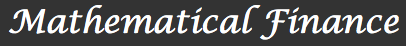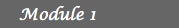Introduction1. The Binomial Model 2. Arbitrage opportunities 3. The First Fundamental Theorem  of Asset Pricing 4. Financial Derivatives 5. Hedging 6. Solutions7. Dividend payments 8. Families of risky assets 9. Time dependent interest rates  and rates of return 10. Short-selling prohibition 11. Multi-factor models 12. SolutionsIntroduction

Throughout these lessons we present an introduction to the main concepts and results of Mathematical Finance, a discipline of Applied Mathematics that has a well-defined theoretical structure worthy of explanation.Louis Jean-Baptiste Alphonse Bachelier: Founder of Mathematical Finance
Mathematical Finance is the branch of mathematics that studies financial markets, which are mechanisms to trade financial securities such as for example stocks or bonds. Stock markets date back to at least 1531 when one was started in Antwerp, Belgium. Mathematical modeling of financial markets by using probability however only came hundreds of years later with the publication in 1900 of Louis Bachelier's thesis titled The Theory of Speculation. Bachelier's work was controversial and generated a hostile response from the Paris Mathematical Community, including his instructor Henri Poincaré, who considered the thesis' topic remote from the ones used to be treated by candidates at the time. Now we realize that Bachelier basically introduced Brownian motion five years earlier than Albert Einstein.

It was only until the 1950's that statistician Savage tipped off economist Paul Samuelson about Bachelier's work. In his 1965 article Samuelson made further developments to Bachelier's initial theory by modeling stock prices according to geometric Brownian motions. After Samuelson's work, developments in Mathematical Finance are closely related to advances in the theory of Stochastic Integration. For instance, the celebrated Robert Merton's Black-Scholes formula for pricing European options is derived by using Itô's stochastic calculus. Also, the results of Paul Meyer and the Strasbourg School on general semimartingale theory proved themselves of great value to model and understand more complicated financial markets. In the subsequent years the topic has attracted the attention of a large number of researchers from different areas such as Economics, Mathematics and Statistics. For a more detailed history of the topic we refer the reader to a recent article by Professors Jarrow and Protter from Cornell University titled "A short history of stochastic and mathematical finance: The early years".

Module 1
In order to avoid the use of some technical tools of Probability and Stochastic Calculus needed to understand the general theory, we will introduce all the concepts by using The Binomial Model, a simple model that reveals clearly the beauty of the subject.
Module 2
The binomial model or CRR model considered in module 1 allowed us to introduce some of the main concepts in mathematical finance. Namely, arbitrage opportunities, risk neutral measures, contingent claims, financial derivatives, hedging strategies and complete markets. However, this model is very simplistic and it is not a good model for real financial markets. There are many aspects that have to be considered in order to achieve a more realistic modeling of financial markets. Among them are, dividend payments, enlargement of the market by considering more risky assets, time dependent riskless bond interest rates and rates of return of the risky asset, constraints over the set of strategies and more general rates of return over the risky assets. In this module we will briefly explain thorough some examples how some of the characteristics of the market change after considering these factors.